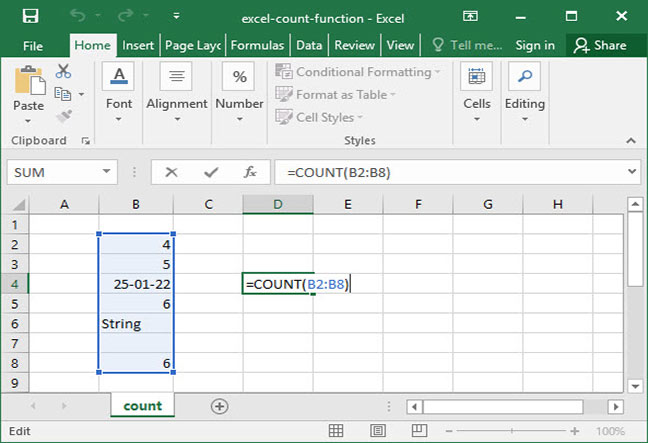# How To Use Excel's COUNT Function

Excel's COUNT function is one of the most powerful tools to help speed up your work and create flexible, dynamic worksheets. Simply put, the COUNT function allows you to count the number of occurences of a numerical value in a range. Let's take a look at how it works.

## The basic COUNT function

The syntax of the COUNT function is very simple:

`=COUNT(value_or_range, value_or_range_2 (optional)...)`

Simply put, COUNT will take all its inputs and spit out the number of inputs that are numerical values (rather than strings of text, blank cells, or errors).

Let's take a look at a simple example of COUNT in action:

`=COUNT(5, 9, 10)Output: 3`

The above formula outputs the number 3, because there are 3 numerical arguments contained within the COUNT function.

Here's another, slightly more complicated, example — in which we introduce both errors and strings of text into the mix:

`=COUNT(5, "Seven", 10, #N/A, 45)Output: 3`

This formula also outputs 3, because only 3 of the arguments provided are numerical.

## COUNT with a range

COUNT can also be used with a range as an input, like so:`=COUNT(B2:B8)Output: 5`

The above formula outputs 5, because five of the cells included in the input range contain numerical values. Note that Excel treats the date, 1/25/2022, as a number. The cell that contains the string, "String", as well as the blank cell, are excluded from the count.

COUNT treats dates as numerical values, because its number formatting stores dates as integers. Try changing the number format on your date cell to "Number" to see what we mean!

## Practical applications of Excel's COUNT function

COUNT has many practical applications within real spreadsheets. For example, you could use it to:

• Count the number of student test scores that were recorded in a given period;
• Count the number of days that a stock price was recorded on the market;
• Find the amount of days for which revenue or profitability data is available in a time series; and
• Count up numerical survey results and control for non-responses.

Once you've mastered COUNT, try moving on to our tutorial on the COUNTA function, which will allow you to count cells that contain any value, including text.

Questions or comments on the above? Let us know in the Comments section below!

## Save an hour of work a day with these 5 advanced Excel tricks

Work smarter, not harder. Sign up for our 5-day mini-course to receive must-learn lessons on getting Excel to do your work for you.

• How to create beautiful table formatting instantly...
• Why to rethink the way you do VLOOKUPs...
• Plus, we'll reveal why you shouldn't use PivotTables and what to use instead...